# Team:KUAS Korea/Model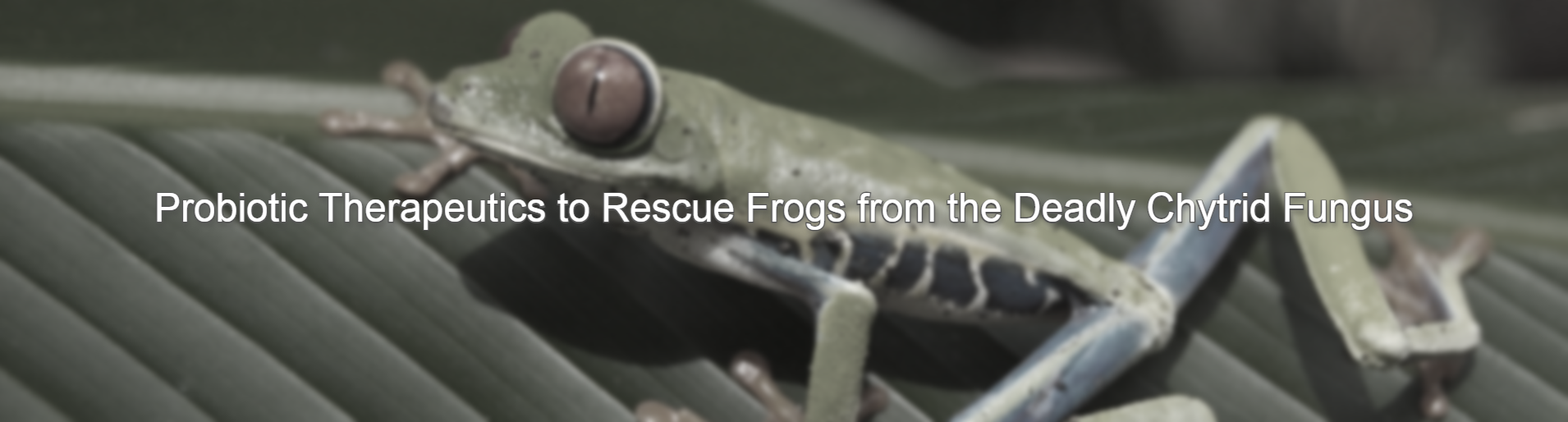# Modeling

## Introduction

We have devised a model that allows frogs to effectively respond to B.dendrobatidis by allowing the design E.coli to produce Violacein when frogs are exposed to B.dendrobatidis in normal life. Therefore, we selected the presence of light and the IPTG emitted after detecting the chitin of fungi as input to output that produces violacein. In order to model to produce violacein when both of these conditions are satisfied, the light induction model produces violacein A and the IPTG induction model produces violacein B,E, so that complete violacein is formed only when both conditions are satisfied. Through the two modeling, we checked how effectively the violacein A, B, and E can be produced under each condition.

## 1. Light induction Violacein modeling

### Model overview

The key point of the light induction model is to effectively produce violacein A when exposed to blue light. Therefore, we selected YF1/FixJ as an optogenetic system for blue light, but YF1/FixJ inhibits expression when exposed to blue light, so we add cI repressors and pRlam promoter regulated by cI repressors as an inverter. Finally, light induction model express violacein A progresses when exposed to blue light.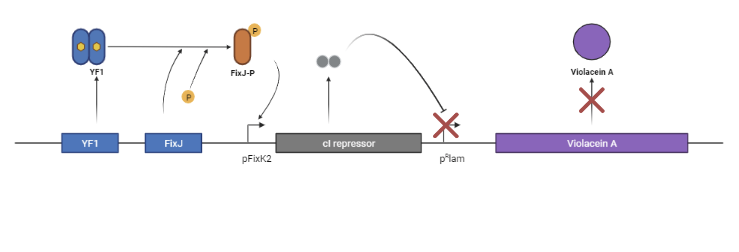The first figure shows the repressing violacein A when blue light is absent or weak.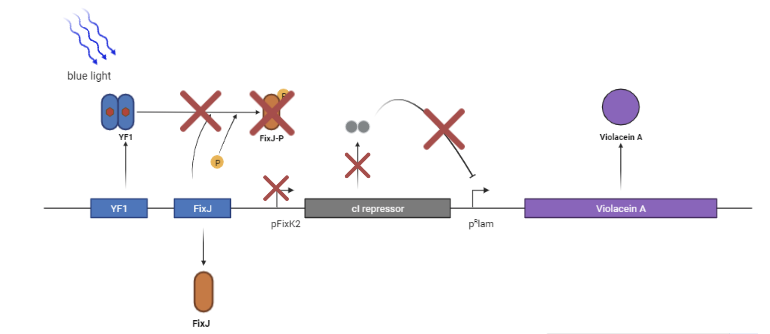the second figure shows the production of violacein A when exposed to blue light.

### ODEs of light induction violacein model

The key point is that YF1 constitutes dimer, and YF1 monomer has D-form and L-form, respectively. Since L-form is formed when exposed to light, the intensity, I value, was reflected. In addition, it is only when dimer is DD form that FixJ can be phosphorylated.
Our model was developed upon the model created by IISER-Pune-India's project in 2019.
The ODEs for light induction violacein model is as below: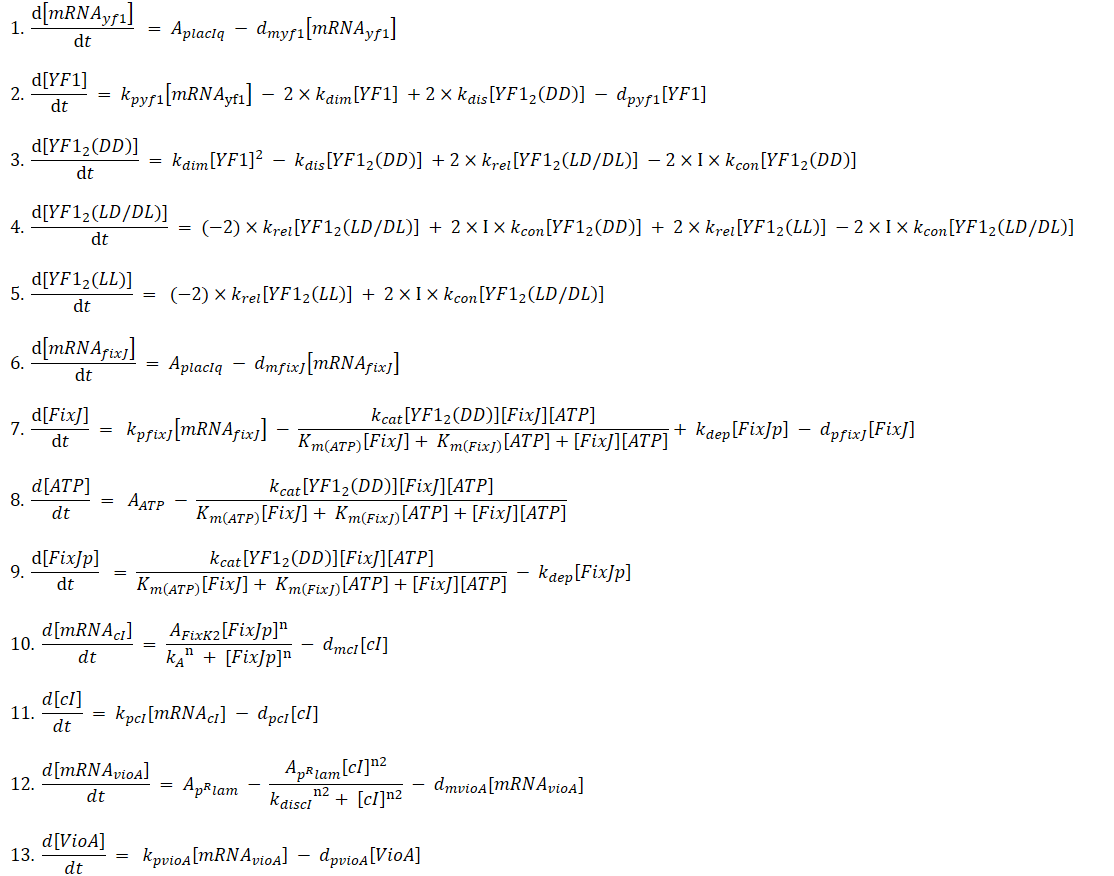### Results

#### 1) No Blue light (I=0)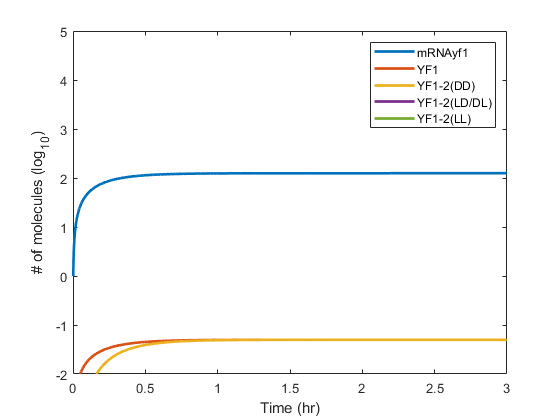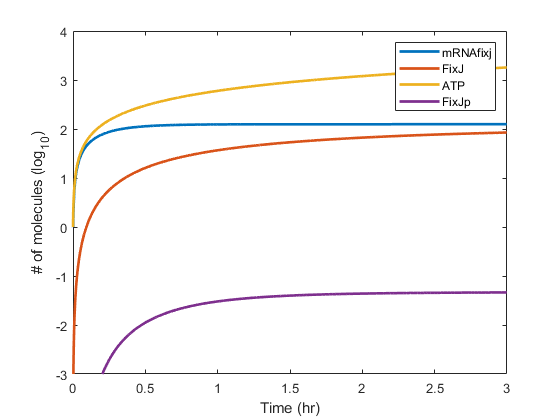When I=0, as we expected, YF1 dimer formed a DD form capable of phosphorylation of FixJ, and FixJ convert to FixJp.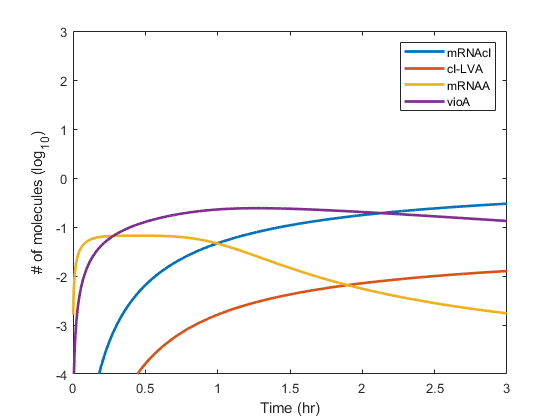If we look at the violacein A production graph, we can see that cI repressors takes some time to act as repressors of the pRlam promoter, so it initially produces some violacein A and eventually decreases.

#### 2) Small intensity of Blue light (I=1)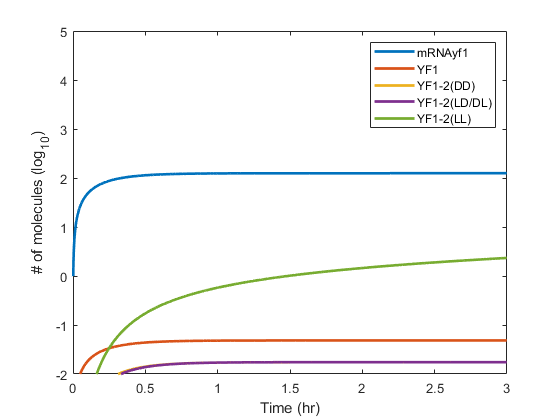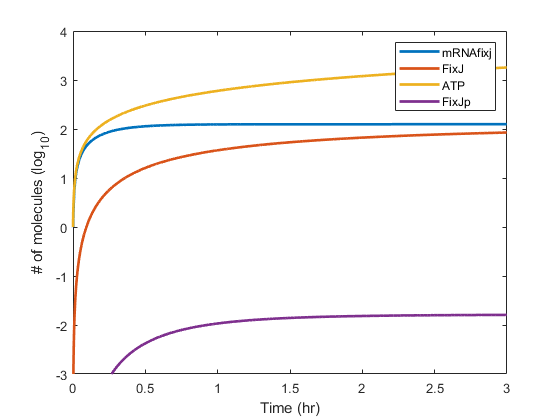When I=1, since YF1 dimer slightly forms DD form, FixJ is phsophorylated less than when I=0.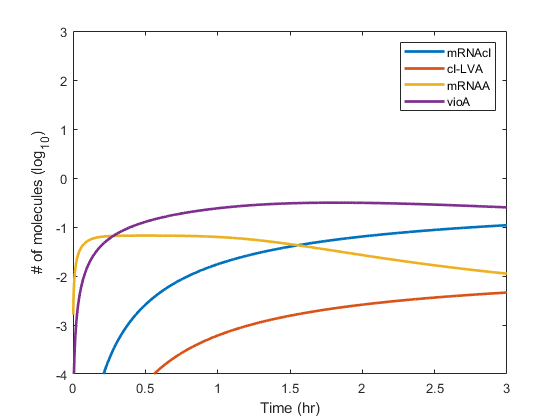If you look at the violacein A production graph, it is similar to the situation when I=0.

#### 3) Large intensity of Blue light (I=100)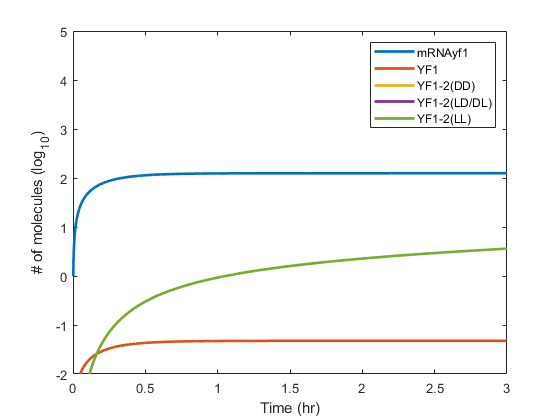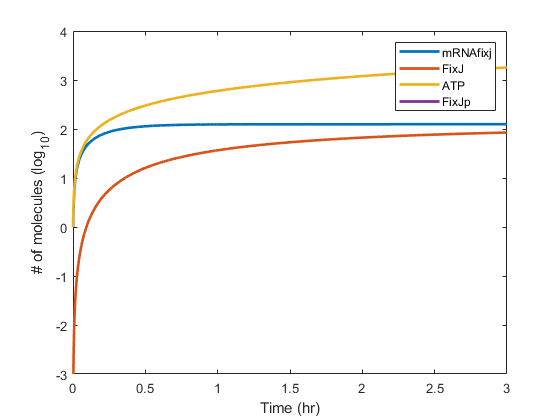When I=100, since the YF1 forms the L-form, the phosphorylation of FixJ is no longer progressed, and thus the production of cI repressors is repressed.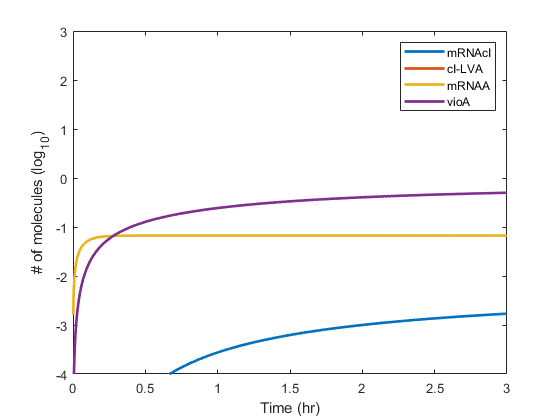There is a large difference in the production of volacein A over time compared to I=0, I=1. When I=100, the production of violacein A proceeds normally.
In genral considering 1), 2), and 3), it can be seen that the light induction model we designed produces Violacein A more effectively than when blue light is present.

### Parameters & matlab codes

Model_Light_induction.zip
parameters.xlsx

## 2. IPTG induction Violacein modeling

### Model overview

We chose to detect chitin, which is mainly present in the fungi cell structure, to detect fungi specifically. This detection of chitin assumes that E.coli produce IPTG in the chitin detedction system mentioned in design and is designed to produce violacein B,E when fungi is detected by controlling the expression of violacein B,E according to the concentration of the IPTG.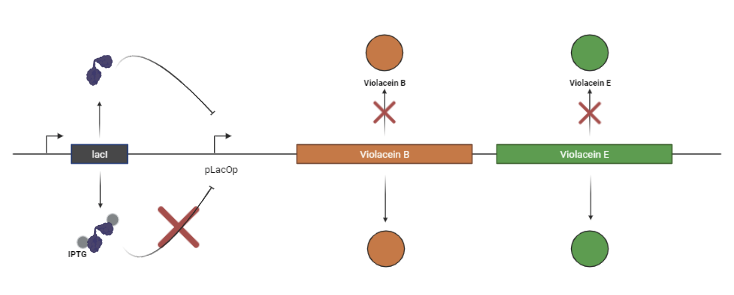This figure schematically shows the production of violacein B,E according to IPTG.

### ODEs of IPTG induction violacein model

The main difference between complexity and simplification is whether the binding between LacOp promoter and LacI was directly used in the ODEs. In addition, simulation reflected the binding of LacI and IPTG into dimers by applying hill coefficient while introducing hill function in equation 5 and 7 after assuming that the combination of LacI and IPTG is monomers. In contrast, complexity considered the combination of IPTG-LacI as a dimer along with the diffusion of IPTG.
Our model was developed upon the model created by Imperial College London’s project in 2009 and the article "Comparison of Deterministic and Stochastic Models of the lac Operon Genetic Network"
The ODEs for IPTG induction model is as below:

#### Complexity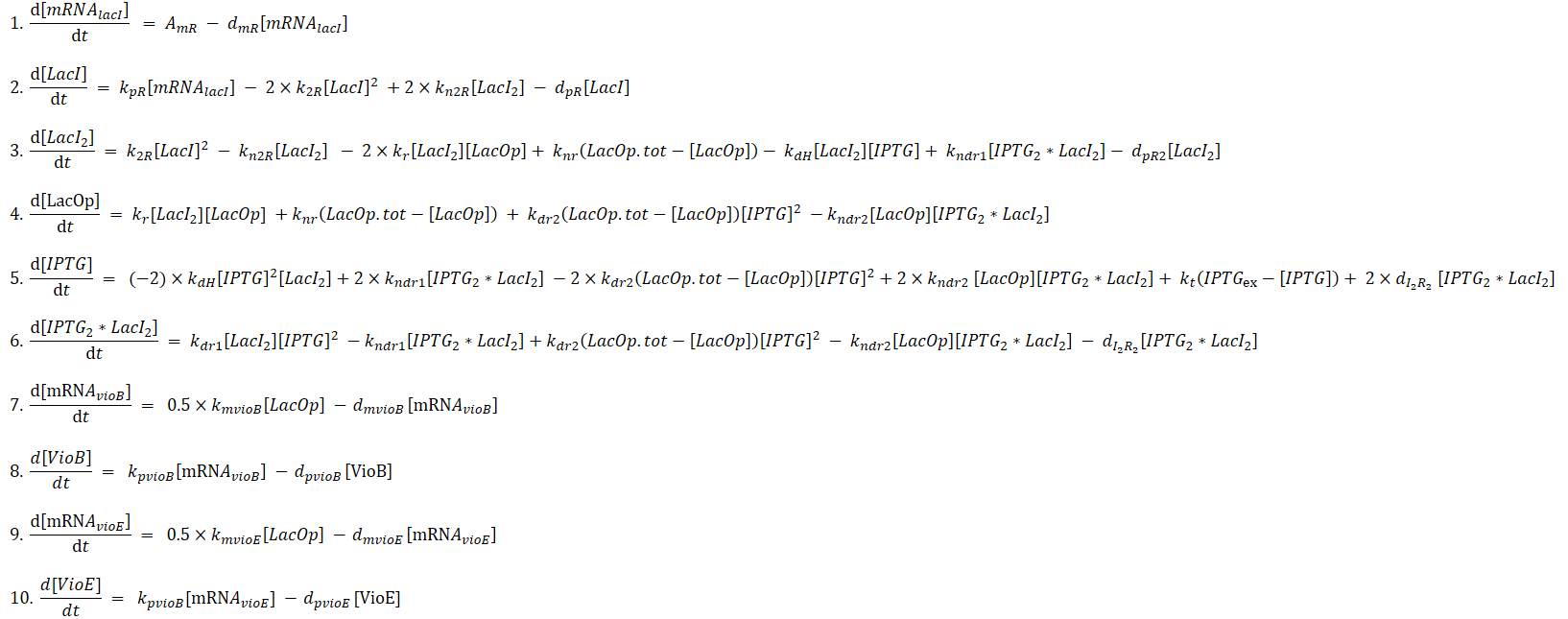#### Simplification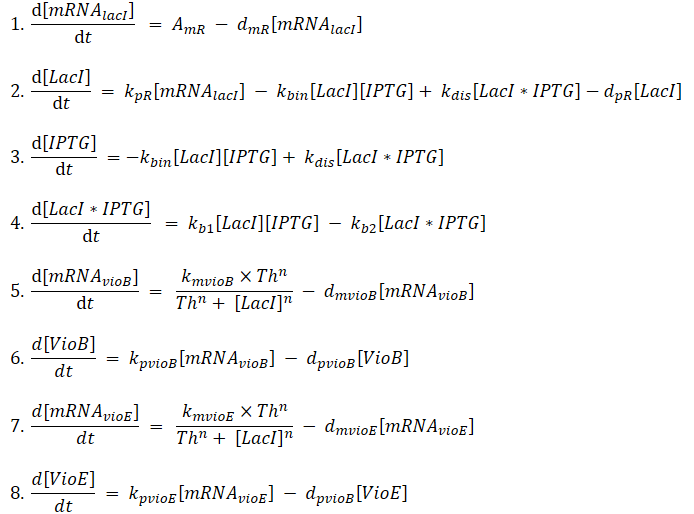References
1. MichailStamatakis et.al., "Comparison of Deterministic and Stochastic Models of the lac Operon Genetic Network",Cell Press, vol.96,(3), 2009, pp.887-906
2. https://openwetware.org/wiki/IGEM:IMPERIAL/2009/M1/Modelling/LacI-IPTG

### Results

#### Complexity

Complexity did not show significant results. As the concentration of IPTG increases, the production of violacein B and E should also increase, but the concentration of violacein B and E showed constant values. We thought that the cause of these results was the application of many factors to modeling, and devised a simplification model to simplify.

#### Simplification

##### 1) [IPTG]init=10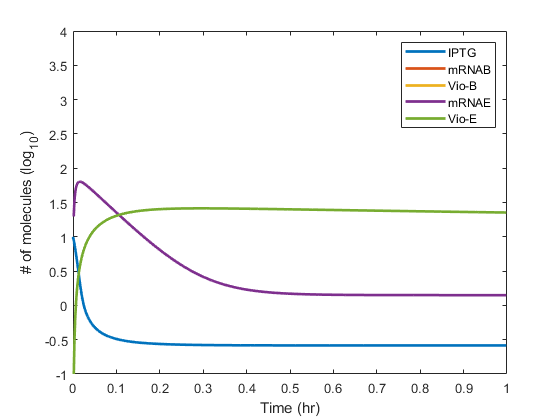##### 2) [IPTG]init=100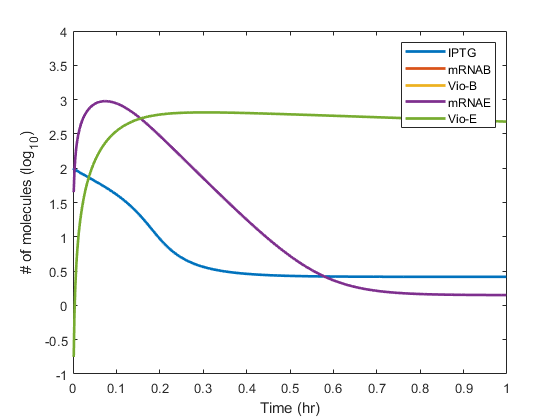Unlike Complexity, as the concentration of IPTG increases, the production of violacein B,E increases.

Considering the above two results, we can see that the production of violacein B and E increases as the IPTG concentration increases through the simplification model designed by us. This means that when chitin is detected, the production of violacein B and E becomes up-regualted.

### Parameters & matlab code

Model_IPTG_induction.zip
parameters.xlsx

## 3. Kill switch construct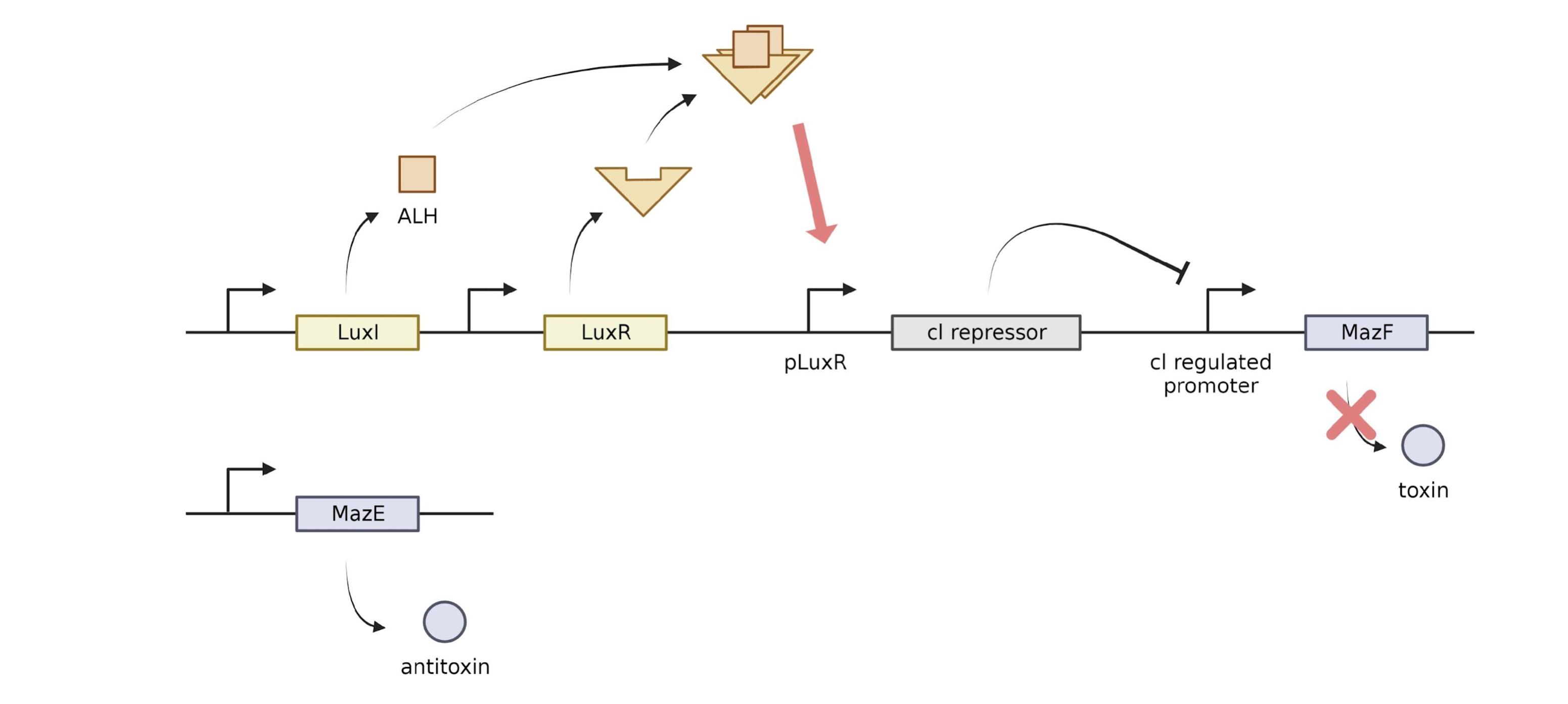image Above is a diagram of our quorum sensing Kill switch. The system is intended to produce the toxin MazF when a cell detaches from the colony and does not recieve enough LuxR/HSL dimers.

### cI at different HSL/LuxR concentrations

In order to know understand the amount of protein(cI repressor) produced by the lux promoter at each transcription factor (HSL/LuxR dimers) levels, we used the Hill equation to plot the graph. For more information about the derivation of the Hill function, see Contributions.

The Matlab code is as below

TF_vs_cl.zip

The result is as below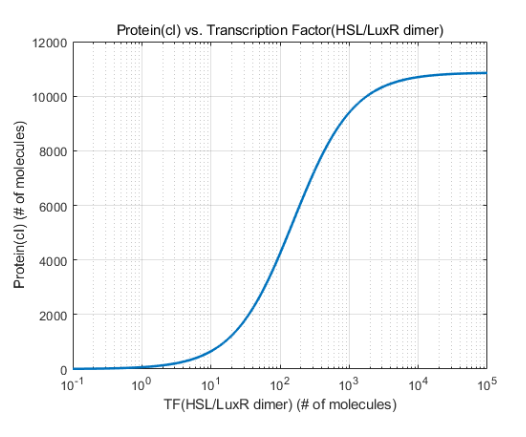The graph tells us that transcription factors(HSL/LuxR dimer) should be in the range of 10~10^4 molecules, and that half the maximum induction is achieved around 100 molecules.

### Construct selection

Our initial design of the kill switch comprised of the J23100 promoters and B0034 RBS constitutively expressing both LuxI and LuxR. However, we were not sure if or design would achieve the proper induction at high densities. So, we created ODE models to evaluate the performance of constructs containing different combinations of promoters and RBSs. We created a single cell model to account for cells that have fallen off of the colony and a high density model for cells with in a colony. Constructs with low HSL/LuxR dimers in the single cell model and high HSL/LuxR dimers in the high density model would be chosen as our improved design. First, when a single cell remains free in the environment, it would be reasonable to consider the external HSL as 0. Internal HSL concentration would be always higher than external HSL, so no HSL would return back into the cell. Thus, in this single cell model, external HSL concentration is fixed to 0.

Our model was developed upon the model created by UPV Valencia's project in 2018 (1). The ODEs for the single cell model is as below: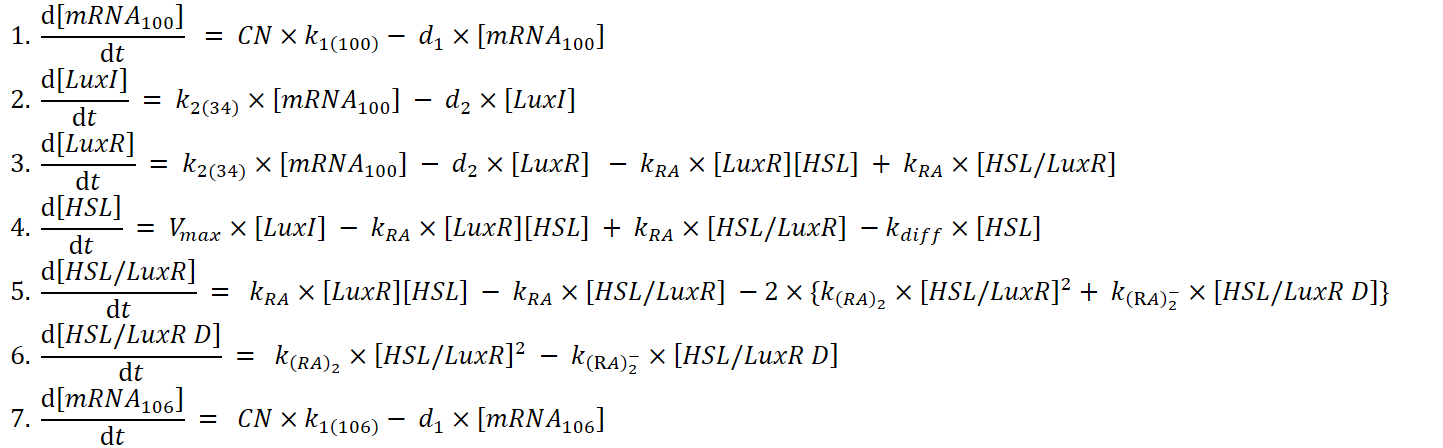Model Single Cell The code is as below:

SingleCellMatlabModel.zip

The results are in the word file below. In the file, the constructs are indicated in the form of R10034_I10632 which means luxR has J23100 as a promoter and B0034 as a RBS, while luxI has J23106 as a promoter and B0032 as a RBS.

Results_SingleCell.docx

The only constructs that had dimers below 10^2 was R10632_I10032 and R10632_I10632.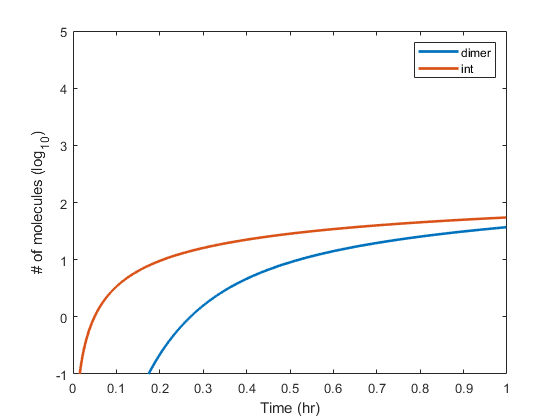HSL/LuxR dimers(dimer) and internal HSL(int) levels for the construct R10632_I10032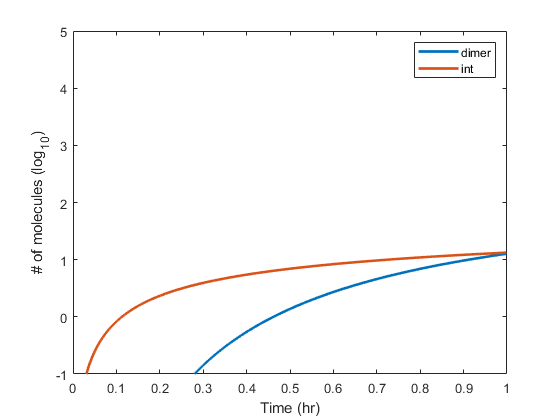HSL/LuxR dimers(dimer) and internal HSL(int) levels for the construct R10632_I10632

### High density culture: closed 1nL env, cells 1(dark color) ~ 1e5(bright color)

When cells are in high density, it would be fairly reasonable to imagine a region homogenous in HSL concentration. So in the high density model, we considered a nL of closed volume. When cells produce HSL, it will start to accumulate and at some point, HSL will flow back into the cell. The ODEs used in the model is as below.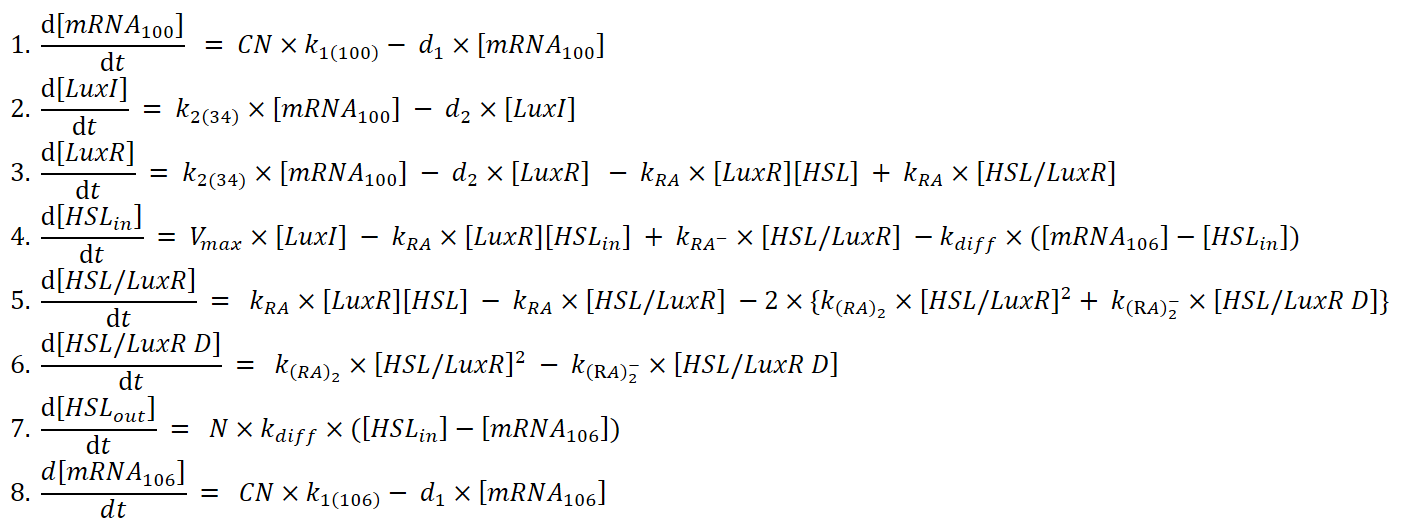Using the ODEs above, we plotted 5 graphs, each corresponding to 1 ~ 10^5 cells/nL, for each constructs. The file below contains the Matlab models used for our modeling.

HIghDensity.zip

The results are in the word file below.

Results_HighDensity.docx

Among the different constructs, the only construct with promising modeling results was the construct with J23106 B0032 which showed around 10^1.5 dimers in at 1cell/nL and 10^2.7 dimers at 10^5cells/nL.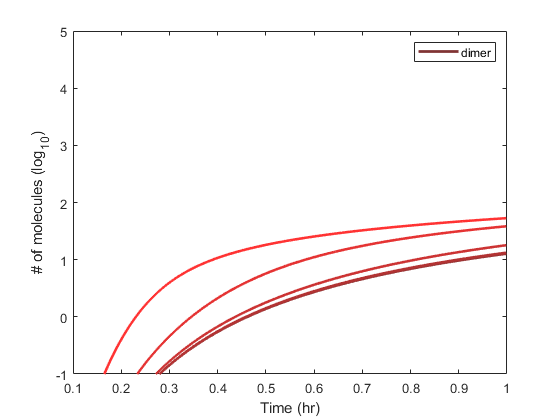The graph above shows the internal(int) and external(ext) HSL in molecules.The graph above shows the number of HSL/LuxR dimers over an hour.

Therefore, our final design will use J23106 & B0032 for both luxR and luxI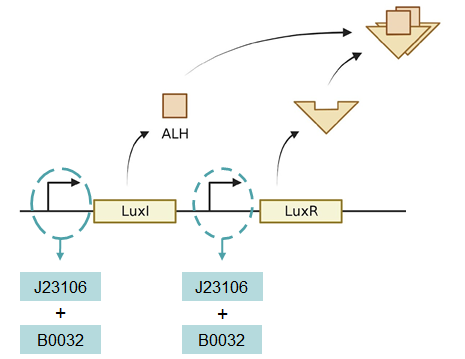KUAS

Korea University

In-Geol Choi

South Korea

Tel: +82 10 3103 7332